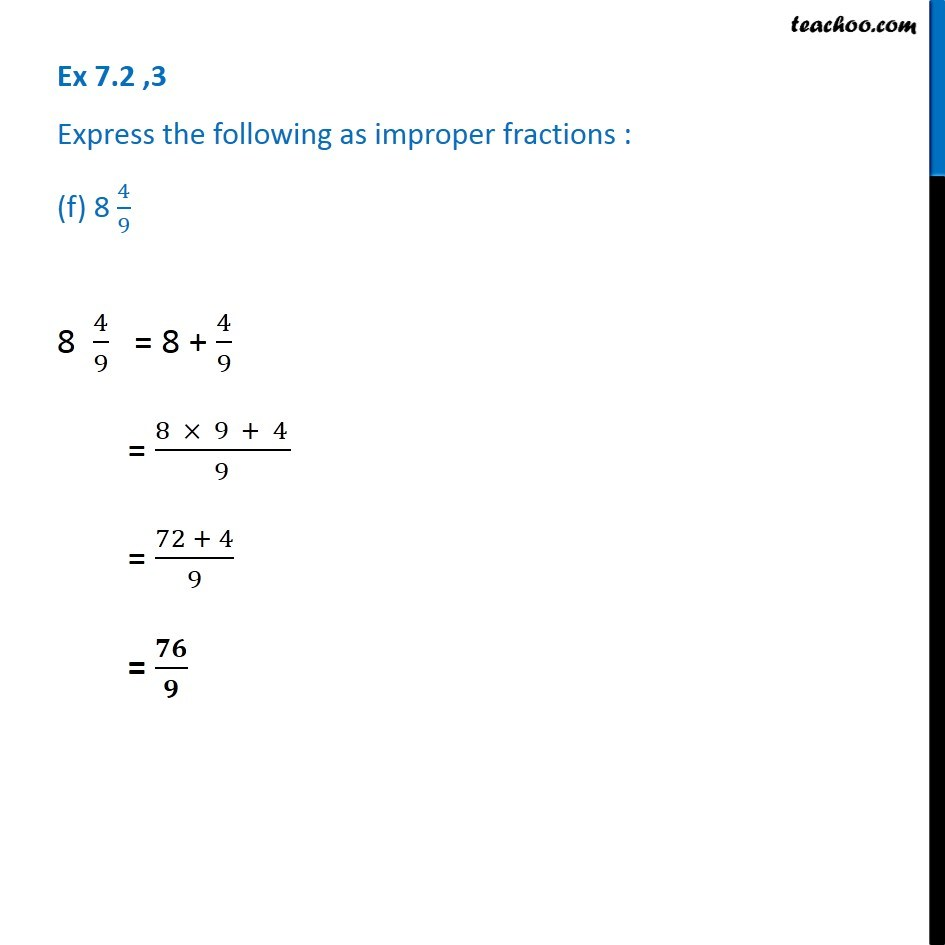1. Chapter 7 Class 6 Fractions
2. Serial order wise
3. Ex 7.2

Transcript

Ex 7.2 ,3 Express the following as improper fractions : (f) 8 4/9 8 4/9 = 8 + 4/9 = (8 × 9 + 4)/9 = (72 + 4)/9 = 𝟕𝟔/𝟗